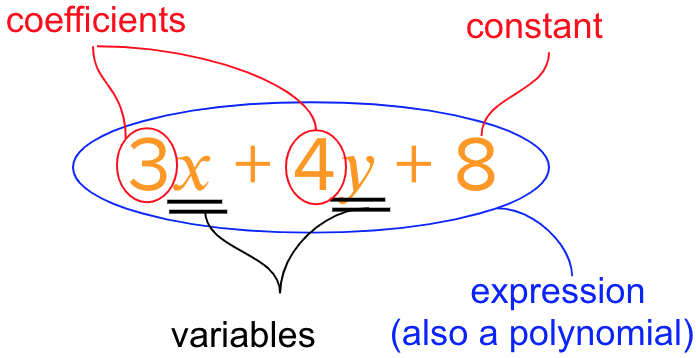# Algebraic Expression

Go back to  'Algebra'

## Basics of Algebra

Here’s a quick glossary and definitions of Algebraic terms:The image above shows a basic equation. This equation is similar to problems in ordinary mathematics.

x and y are variables with 3 and 4 being its respective coefficients. Let’s take a simpler equation:

x+6=9

The question that needs to be answered is “How to solve equations?”

Think of a scale, we keep objects on one side of the scale and weights on the other side to counterweight the object. The same principle is followed here, except instead of weights we use variables and constants.

x+6=9

x+6-6=9-6

x=3

By subtracting 6 on both the sides, we get x=3. The operation needs to be done on both sides of the equation.

x+6=2x+3

x+6-3=2x+3-3

x+3=2x

x+3-x=2x-x

3=x OR x=3

Identifying and using coefficients

The coefficient of a variable is the number which the variable is being multiplied by. Some rules for using coefficients:

• If the variable has no visible coefficient, then it has an implied coefficient of 1

• If the variable only has a negative sign, then the implied coefficient is -1

x+10=3x+6

x+10-6=3x+6-6

x+4=3x

x+4-x=3x-x

4=2x OR 2x=4

Imagine that three friends bought a cake. The total worth of the cake is Rs.30. To determine what the share of each friend is, simply divide the total amount by 3:

30/3=10

Thus each friend has contributed Rs.10. Similarly we can do it to find the value of x in the equation. However, instead of dividing by the number of friends, we divide by the coefficient of the variable. Since we determined that the coefficient of x is 2, we divide each side of the equation by 2:

2x/2=4/2

x=2

## Important Topics

Learn from the best math teachers and top your exams

• Live one on one classroom and doubt clearing
• Practice worksheets in and after class for conceptual clarity
• Personalized curriculum to keep up with school

0